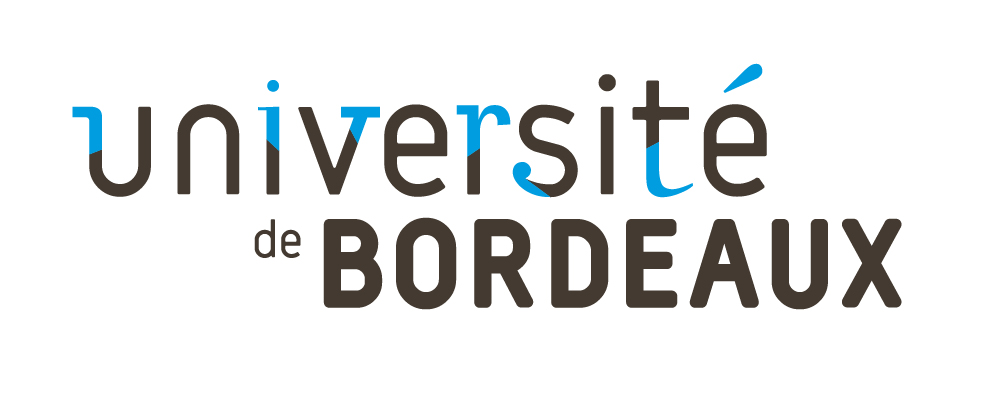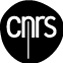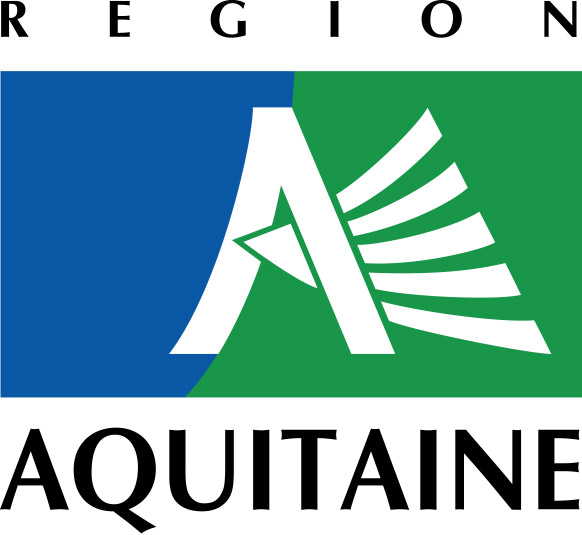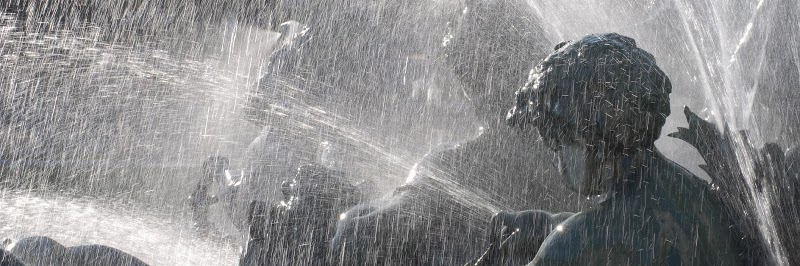Bordeaux Graph Workshop
Bordeaux, November 19-22, 2014.
Enseirb-Matmeca - 1 Avenue du Docteur Albert Schweitzer, 33600 Talence
 > BGW 2014 is the third edition of Bordeaux Graph Workshop. Organized by the "Graphs and Applications" group.Detailed program

### Abstracts of plenary talks

Induced cycles and coloring - Maria Chudnovsky

The Strong Perfect Graph Theorem states that a graph and all its induced subgraphs have equal clique number and the chromatic number if and only if the graph contains no induced odd cycle of length at least five, and no complement of one. But what happens if only some induced cycles (and no complements)are excluded? Gyarfas made a number of conjectures on this topic, asserting that in many cases the chromatic number is bounded by a function of the clique number. In this talk we discuss recent progress on some of these conjectures. This is joint work with Alex Scott and Paul Seymour.

The Firefighter Problem: A survey of directions, results, and problems - Gary MacGillivray

Imagine that a fire breaks out at one or more vertices of a graph, and at each time interval spreads to all neighbouring vertices that have not been protected so far. This is the general setup for the Firefighter Problem, which has attracted considerable attention since being introduced by Hartnell in 1995. We shall survey various directions that have been pursued, and results that have been obtained. Examples include whether the fire can be contained on infinite grids, algorithms, and complexity theorems. Open problems and possible directions for future research will also be discussed.

Discrete isoperimetric problems - Oriol Serra

The isoperimetric problem for graphs asks for the cardinality of the minimum (edge or vertex) boundary among sets of vertices with given cardinality. An ordering of the vertex set of a graph is isoperimetric if its initial segments are optimal sets for the isoperimetric problem. The classical theorems of Kruskal-Katona and Harper show that the n-cube admits an isoperimetric order. In the talk we will discuss a local-global principle which extends analogous results for certain classes of cartesian products of graphs and provides simple proofs for several known results. Algorithmic aspects concerning the existence of a set of nested solutions for graphs with bounded treewidth will be also addressed.

List colorings of planar graphs - Margit Voigt

Local irregularity - Mariusz Wozniak

A locally irregular graph is a graph whose adjacent vertices have distinct degrees. We say that a graph G can be decomposed into k locally irregular subgraphs if its edge set may be partitioned into k subsets each of which induces a locally irregular subgraph in G. We characterize all connected graphs which cannot be decomposed into locally irregular subgraphs. These are all of odd size and include paths, cycles and a special class of graphs of maximum degree 3. Moreover we conjecture that apart from these exceptions all other connected graphs can be decomposed into three locally irregular subgraphs. We also investigate a total version of this problem, where in some sense also the vertices are being prescribed to particular subgraphs of a decomposition. The both concepts are closely related to the known 1-2-3 Conjecture and 1-2 Conjecture, as well as to other similar problems concerning edge and arc colorings of graphs and digraphs, respectively.

Online list colouring of graphs - Xuding Zhu

The on-line colouring game on a family F of graphs is played by two players: Presenter and Algorithm. In each round, Presenter add one new vertex v to the existing graph, including all edges connecting v to earlier vertices. The resulting graph must be in the family F. Algorithm colours v with a colour not used by any of its existing neighbours. The n-round) on-line chromatic number of F is the least number of colours sufficient for Algorithm to play the game for n-rounds. Equivalently, it is the maximum number of colours Presenter can force the Algorithm to use in n-rounds. It was proved by Lovasz, Saks and Trotter that the (n-round) on-line chromatic number of bipartite graphs is at most 2 log n. A recent result of Bianchi shows that it is at least 1.13746 log n - 0.49887. We improve this lower bound to 2 log n - 10, which differs from the upper bound by an additive constant. It was proved by Kierstead that graphs of odd girth 7 has (n-round) on-line chromatic number O(n^{1/2}), and we give a lower bound and prove that this family of graphs has (n-round) on-line chromatic number Omega(n/((log n)^{1/3})). This is a joint work with Grzegorz Gutowski, Jakub Kozik and Piotr Micek.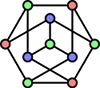LaBRI
Université de Bordeaux
Bordeaux INP
ANR DORSO
Cluster d'excellence CPU
Conseil Régional